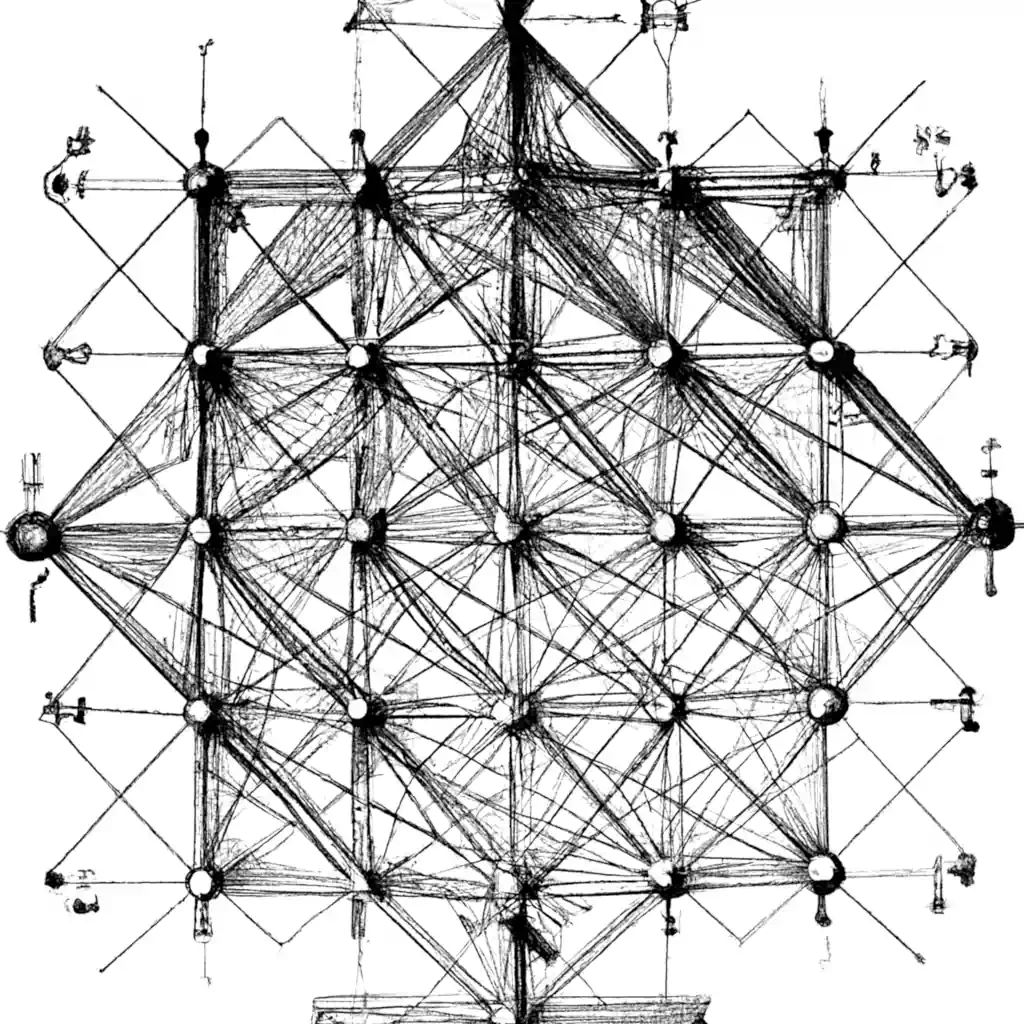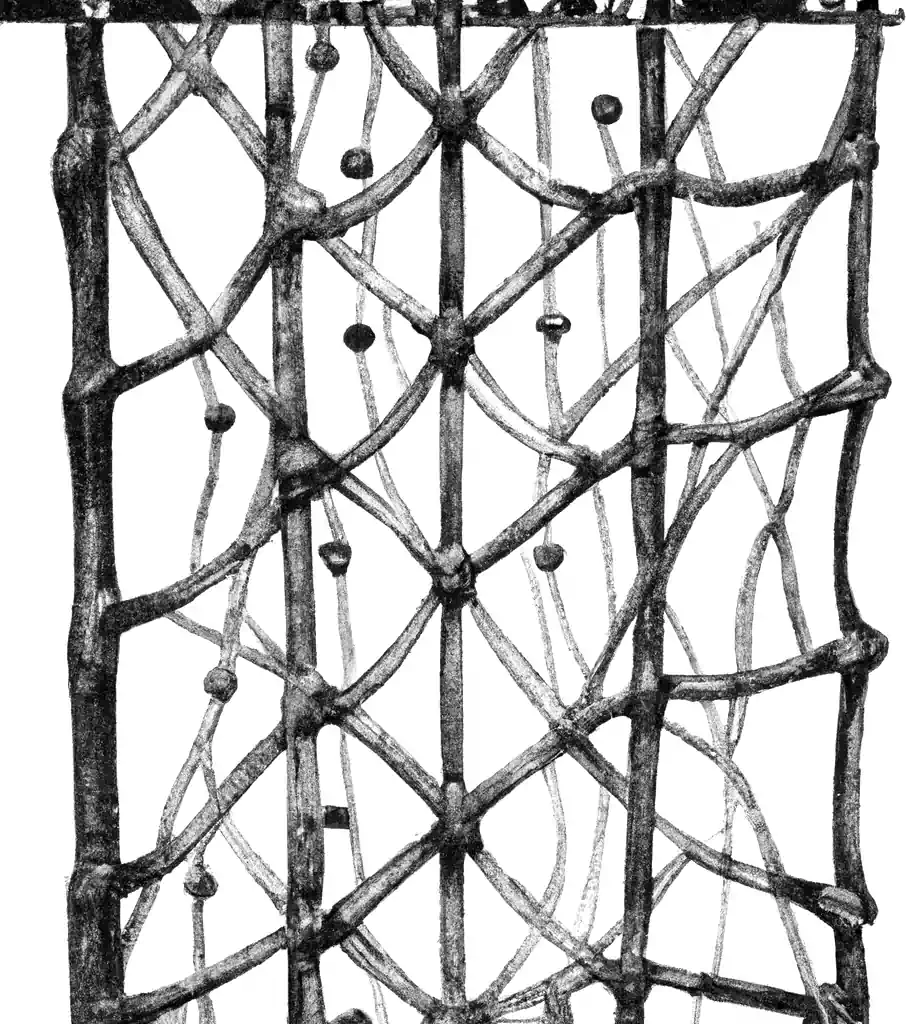# Simulating Gaussian processes on a lattice

### Assumed audience:

ML people

How can I simulate a Gaussian Processes on a lattice with a given covariance?The general (non-lattice) case is given in historical overview in Liu et al. (2019), but in this notebook we are interested in specialising a little. Following the introduction in Dietrich and Newsam (1993), let’s say we wish to generate a stationary Gaussian process $$Y(x)$$ on a points $$\Omega$$. $$\Omega=(x_0, x_1,\dots, x_m)$$.

Stationary in this context means that the covariance function $$r$$ is translation-invariance and depend only on distance, so that it may be given $$r(|x|)$$. Without loss of generality, we assume that $$\bb E[Y(x)]=0$$ and $$\var[Y(x)]=1$$.

The problem then reduces to generating a vector $$\vv y=(Y(x_0), Y(x_1), \dots, Y(x_m) )\sim \mathcal{N}(0, R)$$ where $$R$$ has entries $$R[p,q]=r(|x_p-x_q|).$$

Note that if $$\bb \varepsilon\sim\mathcal{N}(0, I)$$ is an $$m+1$$-dimensional normal random variable, and $$AA^T=R$$, then $$\vv y=\mm A \vv \varepsilon$$ has the required distribution.

## The circulant embedding trickIf we have additional structure, we can work more efficiently.

Suppose further that our points form a grid, $$\Omega=(x_0, x_0+h,\dots, x_0+mh)$$; specifically, equally-spaced-points on a line.

We know that $$R$$ has a Toeplitz structure. Moreover it is non-negative definite, with $$\vv x^t\mm R \vv x \geq 0\forall \vv x.$$ (Why?) 🏗

Wilson et al. (2021) credits the following authors:

Well-known examples of this trend include banded and sparse matrices in the context of one-dimensional Gaussian processes and Gauss–Markov random fields [;Durrande et al. (2019)], as well as Kronecker and Toeplitz matrices when working with regularly-spaced grids .### No comments yet. Why not leave one?

GitHub-flavored Markdown & a sane subset of HTML is supported.